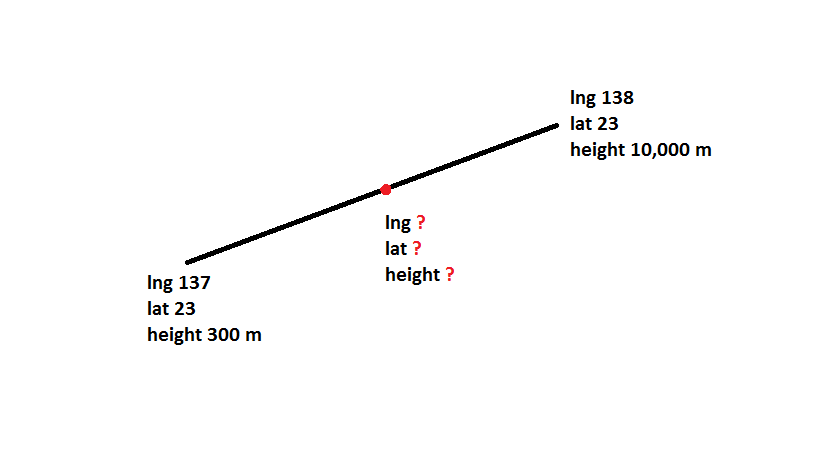# Midpoint in line

Hi,

I have two points. Each is defined by lat/long/height.

I’m drawing a straight line in between them (I’m not concerned about following the surface of the earth, just a straight line is what I want).

Is it possible to calculate the midpoint between the two? Ie. Calculate the lat/long/height of the point that is in the middle of the line?

Thank you,

FidelHi Fidel,

This is a classic graphics math problem. To compute the midpoint between two points A and B, we an compute the vector between them by taking (B - A), normalizing to get the direction vector. Then multiplying this direction vector by magnitude of (B-A) multiplied by the fraction of the way we want to travel between them. Then you can just convert to Cartographic. Here’s the code for this: http://cesiumjs.org/Cesium/Apps/Sandcastle/?src=Hello%20World.html&label=Showcases&gist=d290d6e52cdeec93ac14c8a0aef1a8b6

You can also use linear interpolation to get an intermediate point. Let me know if you want to discuss that method also.

Hope that helps!

• Rachel

This is perfect Rachel! Exactly what I was looking for. Thank you so much.

Could you please let me know about linear interpolation as well? I had been trying with Ellipsoid.interpolateUsingFraction() but I wasn’t getting a height component.